GeeksforGeeks App
Open AppBrowser
Continue

## Related Articles

• RD Sharma Class 10 Solutions

# Class 10 RD Sharma Solution – Chapter 11 Constructions – Exercise 11.3

### Question 1. Draw a circle of radius 6 cm. From a point 10 cm away from its centre, construct the pair of tangents to the circle and measure their lengths.

Solution:

Follow these steps for construction :

Step 1: Construct of 6 cm radius with centre O.

Step 2: Mark a point P, 10 cm away from the centre O.

Step 3: Now join PO then bisect it at M.

Step 4: From the centre M and the diameter PO, construct a circle that will be intersecting the given circle at T and S.

Step 5: Thus join PT and PS.

Further PT and PS are the required tangents.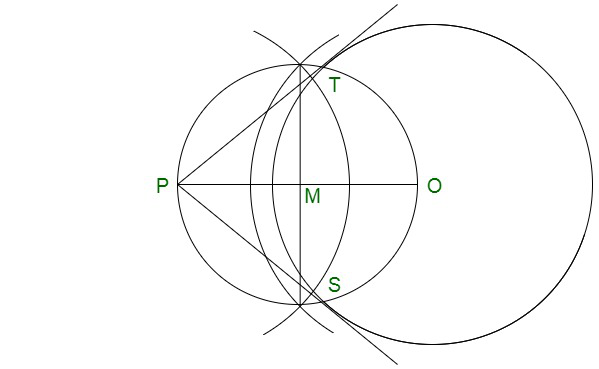### Question 2. Draw a circle of radius 3 cm. Take two points P and Q on one of its extended diameter each at a distance of 7 cm from its centre. Draw tangents to the circle from these two points P and Q.

Solution:

Follow these steps for construction :

Step 1: Construct of radius 3 cm with the centre O.

Step 2: Construct a diameter.

Step 3: Mark two points P and Q on this diameter with a distance of 7 cm each from the centre O, as shown below.

Step 4: Now bisect QO at N and PO at M.

Step 5: From the centres M and N, construct circle on ‘PO’ and ‘QO’ as diameter which intersect the given circle at S, T and S’, T’ respectively.

Step 6: Further join PS, PT, QS’ and QT’.

Therefore, PS, PT, QS’ and QT’ are the required tangents to the given circle.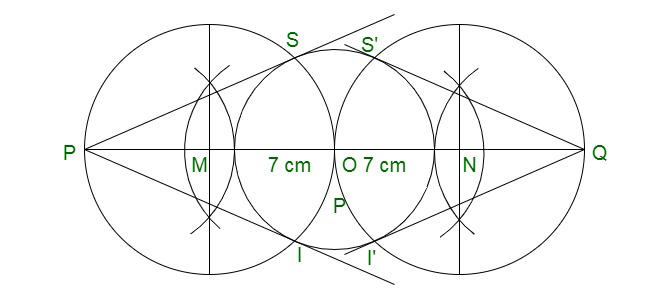### Question 3. Draw a line segment AB of length 8 cm. Taking A as centre, draw a circle of radius 4 cm and taking B as centre, draw another circle of radius 3 cm. Construct tangents to each circle from the centre of the other circle. [CBSE 2013]

Solution:

Step 1: Construct a line segment AB of 8 cm.

Step 2: Draw circles from the centre A and radius 4 cm and with centre B and radius 3 cm.

Step 3: Now bisect AB at M.

Step 4: From centre M and diameter AB, construct a circle which will intersects the two circles at S’, T’ and S, T respectively.

Step 5: Further join AS, AT, BS’and BT’.

Therefore, AS, AT, BS’ and BT’ are the required tangent.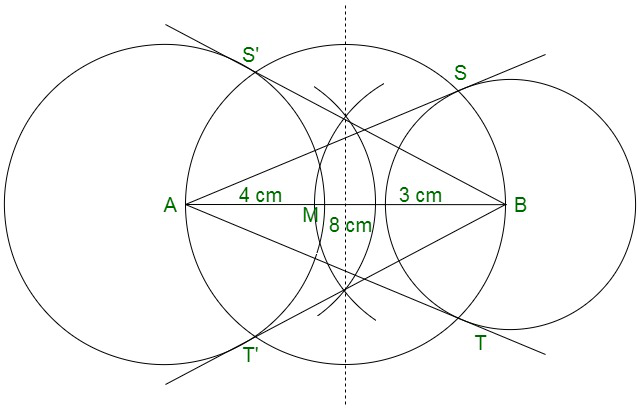### Question 4. Draw two tangents to a circle of radius 3.5 cm from a point P at a distance of 6.2 cm from its centre.

Solution:

Follow these steps for construction :

Step 1: Construct a circle of radius 3.5 cm with centre O.

Step 2: Mark a point P which will be of 6.2 cm from O.

Step 3: Now bisect PO at M and construct a circle with centre M and diameter OP which will intersects the given circle at T and S respectively.

Step 4: Further join PT and PS.

Therefore, PT and PS are the required tangents to circle.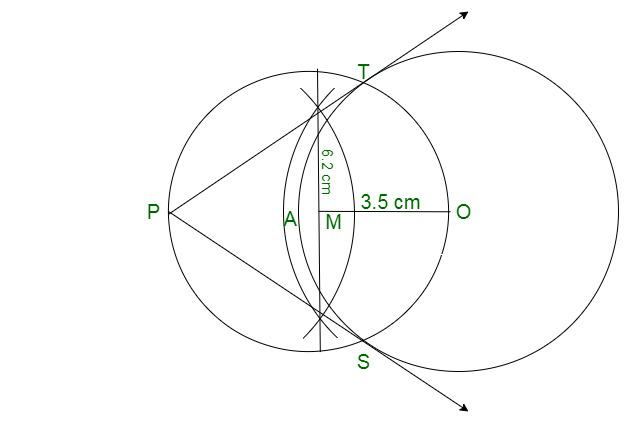### Question 5. Draw a pair of tangents to a circle of radius 4.5 cm, which are inclined to each other at an angle of 45°. [CBSE 2013]

Solution:

At centre the angle is 180° – 45° = 135°

Step 1: Construct a circle of radius 4.5 cm with centre O.

Step 2; Now, at O, construct an angle ∠TOS = 135°

Step 3: Further at T and S draw perpendicular which will meet at P.

Therefore, PT and PS are the tangents which inclined each other 45°.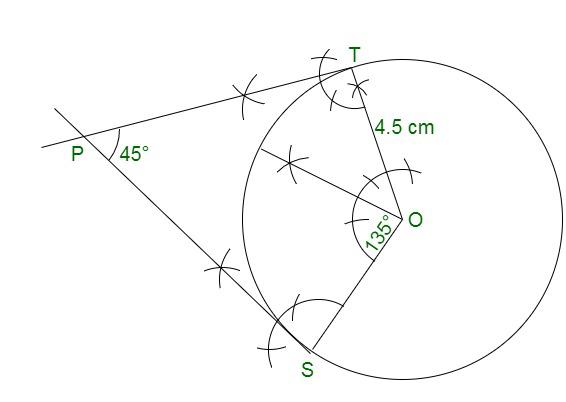### Question 6. Draw a right triangle ABC in which AB = 6 cm, BC = 8 cm and ∠B = 90°. Draw BD perpendicular from B on AC and draw a circle passing through the points B, C and D. Construct tangents from A to this circle.

Solution:

Step 1: Construct a line segment BC of 8 cm

Step 2: From B construct an angle of 90°

Step 3: Construct an arc BA˘ of 6cm cutting the angle at A.

Step 4: Now join AC. Thus, ΔABC is the required A.

Step 5: Construct perpendicular bisector of BC cutting BC at M.

Step 6: Mark M as centre and BM as radius, construct a circle.

Step 7: Mark A as centre and AB as radius, construct an arc cutting the circle at E. Thus, join AE.

Therefore, AB and AE are the required tangents.

Justification:

Given: ∠ABC = 90°

Thus, OB is a radius of the circle.

Therefore, AB is a tangent to the circle.

Also AE is a tangent to the circle.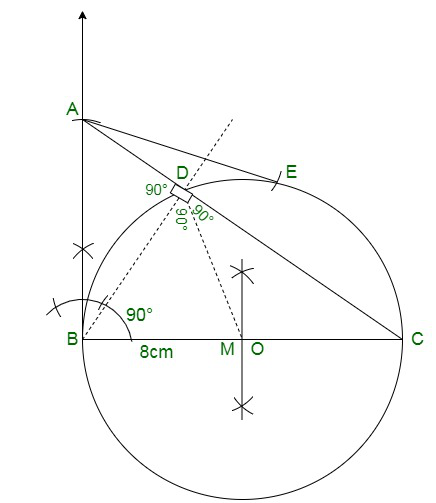### Question 7. Draw two concentric circles of radii 3 cm and 5 cm. Construct a tangent to the smaller circle from a point on the larger circle. Also, measure its length. [CBSE 2016]

Solution:

Given: Two concentric circles of radii 3 cm and 5 cm with centre O.

We have to construct a pair of tangents from point P on outer circle to the other.

Step 1: Construct two concentric circles of radii 3 cm and 5 cm with the centre O.

Step 2: Then, take any point P on outer circle and join OP.

Step 3: Now, bisect OP and let M’ be the mid-point of OP.

Take M’ as centre and OM’ as radius construct a circle dotted which will cut the inner circle as M and P’.

Step 4: Further, join PM and PP’. Therefore, PM and PP’ are the required tangents.

Step 5: After measuring PM and PP’, we find that PM = PP’ = 4 cm.

Actual calculation:

In right angle ΔOMP, ∠PMO = 90°

Therefore,

PM2 = OP2 – OM2   {by Pythagoras theorem i.e. (hypotenuse)2 = (base)2 + (perpendicular)2}

⇒ PM2 = (5)2 – (3)2 = 25 – 9 = 16

⇒ PM = 4 cm

Hence, the length of both tangents is 4 cm.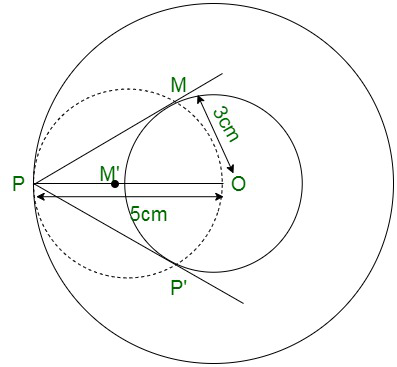My Personal Notes arrow_drop_up
Related Tutorials